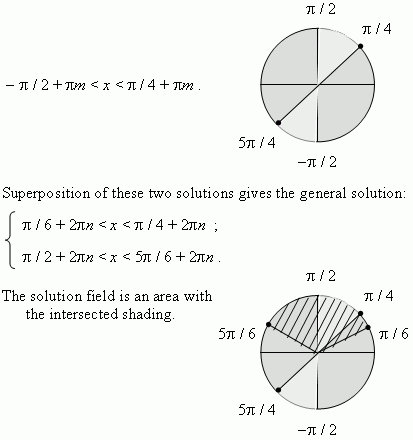# Trigonometric inequalities

At solving of trigonometric inequalities we use the properties of inequalities, known from algebra and also the trigonometric transformations and formulas. Using of the unit circle at solving of trigonometric inequalities is almost necessary. Consider some examples.

E x a m p l e  1 .  Solve the inequality:   sin x > 0.

S o l u t i o n .  Within one revolution of the unit radius this inequality is valid at

0 < x <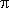. Now it is necessary to add the sine period  2n :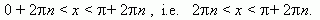E x a m p l e  2 .  Solve the inequality:   sin x > 0.5 .

S o l u t i o n .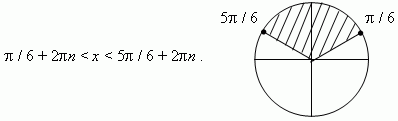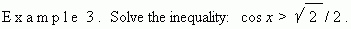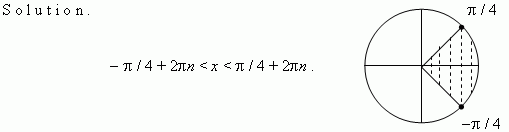E x a m p l e  4 .  Solve the system of simultaneous inequalities: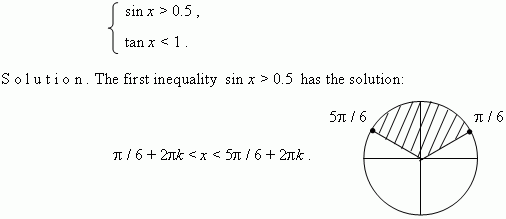The second inequality  tan x < 1  has the solution: